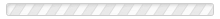• The level of activity where revenues equal total expenses, producing a zero net income; also the point where the contribution margin is said to cover fixed costs
• Costs that arise from an organization's commitment to engage in operations; unavoidable elements like depreciation, rent, insurance, property taxes
• Revenues minus all variable expenses, whether related to production or selling and administration (not to be confused with gross profit)
• (CVP) Analysis focusing on the interplay of pricing, volume, variable and fixed costs, and product mix
• Fixed cost resulting from yearly spending decisions; proper planning can result in avoidance of these costs as necessary (e.g., advertising and training)
• Efficiencies associated with increases in volume
• A total cost that is the same regardless of volume; total cost is constant and per unit cost decreases with volume increases
• A simple means for separating costs into fixed and variable components, based upon the difference between costs at the highest and lowest observed levels of activity
• A complex means for separating costs into fixed and variable components, based upon minimizing the variances between all observations and the resulting assumed cost function
• A cost that has both fixed and variable components
• The level of activity for which assumptions underlying CVP are expected to hold true
• A simplistic mapping of observed data points, where a line is "visually" drawn to represent the estimated cost function
• A cost function that is fixed over a range, and then increases by a measured step to a new level at the next higher increment of activity
• A level of income that is to be obtained; CVP projects activity levels necessary to achieve this benchmark
• A per unit cost that is the same regardless of volume; total variable cost increases with volume increases